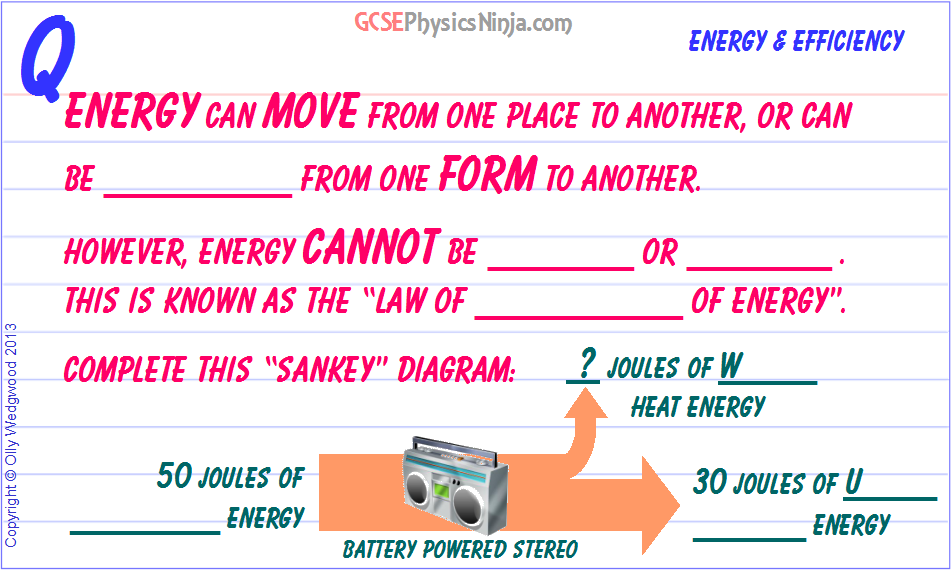# 2. Sankey diagram and energy transfers-

``` ```

## How energy flows can be described in a Sankey Diagram

This is one of the most important physics principles in the universe! Energy can move from one place to another, or it can be transferred (or transformed) from one form to another.

However, energy cannot be created or destroyed. This is a fundamental principle in physics. Energy can always change into another form - it won't just disapper into nothing, and you cannot create energy from nothing.

This is called the Law of Conservation of Energy.

To illustrate this law, we can use a Sankey Energy diagram. The width of each arrow represents the amount of energy. For examples, consider a battery powered stereo.

To start of with, the stereo's batteries supply 50 Joules of chemical potential energy which is then transferred into electrical energy in the wires and circuitry. This 50 Joules is the total energy input.

Then the electrical energy will eventually be transferred into kinetic energy of the vibrating speaker cones and then into the pulse of sound energy which travel to your ears. This output sound energy is 'useful' energy as it's the type of energy we want from a stereo.

Whenever an arrow splits in a Sankey Diagram, it means that energy has transferred to different forms. The widths of the new arrows (new energy forms) must add up to the width of the starting arrow. This is the Law of Conservation of Energy in action.

Some of the input energy will be transferred to heat energy which is 'wasted' - we don't need this type of energy from a stereo. (However, you might want useful heat energy from an electrical heater).

So, if the Sankey diagram starts with 50 Joules of chemical energy, and ends with 30 Joules of sound energy, then the amount of wasted heat energy in the other arrow must be:

50 - 30 = 20 J

Make sure that you can draw a Sankey diagram like this one, taking care that the widths of each arrow represent the amount of energy. Also make sure that your diagram obeys the Law of Conservation of Energy so that the input energy = output energy (both useful and wasted forms)

GCSE Physics Keywords: Sankey Diagram, Law of Conservation of Energy, Useful energy, Wasted energy

Course overview